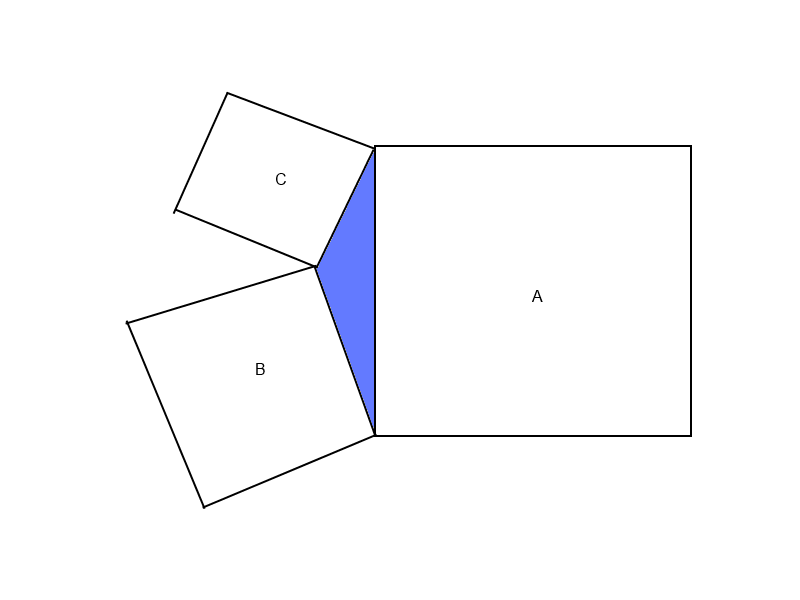# Is it surely Heron?In the figure to the right, the areas of the squares $A, B,$ and $C$ are 388, 153, and 61, respectively.

Find the area of the blue triangle.

×